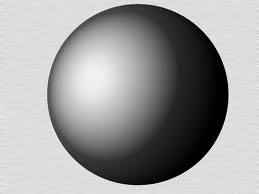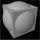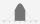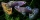# Sphere area

A cube with edge 1 m long is circumscribed sphere (vertices of the cube lies on the surface of a sphere). Determine the surface area of the sphere.

Correct result:

S =  9.4248 m2

#### Solution:We would be very happy if you find an error in the example, spelling mistakes, or inaccuracies, and please send it to us. We thank you!#### You need to know the following knowledge to solve this word math problem:

We encourage you to watch this tutorial video on this math problem:

## Next similar math problems:

• Cube from sphereWhat largest surface area (in cm2) can have a cube that was cut out of a sphere with radius 43 cm?
• Sphere vs cubeHow many % of the surface of a sphere of radius 12 cm is the surface of a cube inscribed in this sphere?
• Fit ballWhat is the size of the surface of Gymball (FIT - ball) with a diameter of 65 cm?
• Surface area of a cubeWhat is the surface area of a cube that has an edge of 3.5?
• SeatHow much m² of fabric do we need to sew a 50cm-shaped cube-shaped seat if 10% of the material we add to the folds?
• Cube surface areaWall of cube has content area 99 cm square. What is the surface of the cube?
• Normal distribution GPAThe average GPA is 2.78 with a standard deviation of 4.5. What are students in the bottom the 20% having what GPA?
• Cube cornersFrom cube of edge 5 cm cut off all vertices so that each cutting plane intersects the edges 1 cm from the nearest vertice. How many edges will have this body?
• Guppies for salePaul had a bowl of guppies for sale. Four customers were milling around the store. 1. Rod told paul - I'll take half the guppies in the bowl, plus had a guppy. 2. Heather said - I'll take half of what you have, plus half a guppy. The third customer, Nancy
• TrigonometryIs true equality? ?
• Sequence 2Write the first 5 members of an arithmetic sequence a11=-14, d=-1
• SequenceWrite the first 7 members of an arithmetic sequence: a1=-3, d=6.
• BlocksThere are 9 interactive basic building blocks of an organization. How many two-blocks combinations are there?
• LineIt is true that the lines that do not intersect are parallel?
• Reference angleFind the reference angle of each angle:
• Today in schoolThere are 9 girls and 11 boys in the class today. What is the probability that Suzan will go to the board today?
• Elimination methodSolve system of linear equations by elimination method: 5/2x + 3/5y= 4/15 1/2x + 2/5y= 2/15